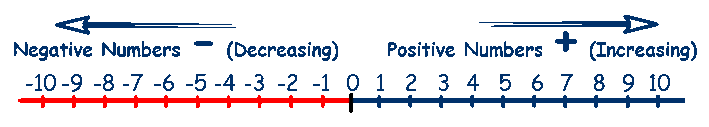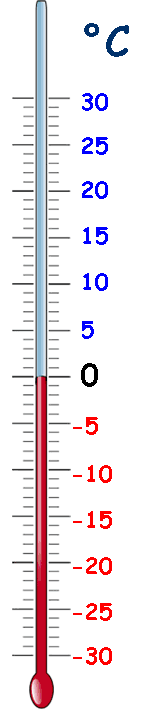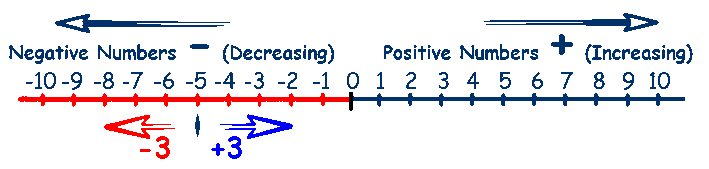# Negative Numbers

## Negative numbers are numbers that are less than zero.

Negative numbers are written with a minus sign in front of them
negative 5 is written as -5, negative 10 is written as -10 etc.

Negative numbers are also called 'minus' numbers (do not confuse this with subtraction).

Numbers that are greater than zero are called Positive Numbers and are
written just as the number (no need to put a + in front).

The main thing to understand and remember is:

and

##The number line above, shows that as positive numbers get larger, their value increases.
6 is larger than 3

But, as negative numbers get larger, their value decreases, -9 is less than -5.This is a difficult concept for children to understand.

I normally relate it to temperature, which is something that they are familiar with and a thermometer is basically a vertical number line.

On a hot summer day, the temperature might be 25°C and at night the the temperature might fall to 15°C.

As the temperature decreases, the numbers decrease
(because so far the numbers have all been positive).

On a cold winter day, the temperature might be 10°C and at night the the temperature might fall to 0°C.

Again as the temperature decreases, the numbers decrease
(because the numbers are still positive).

On a really cold winter day, the temperature might be 0°C and at night the temperature might fall to -10°C.

Now as the temperature decreases, the numbers increase.
(because the numbers are now negative).

It is common for children to think that -10 has a greater value than -5, because the 10 is higher than 5.

These worksheets from Mathsblog should help:

Another way of explaining this is in terms of money in a bank account - if you have -10 pounds in your account, you are overdrawn and owe the bank £10.

## Adding and Subtracting Positive and Negative Numbers

Adding positive numbers is straightforward .... 3 + 4 = 7
A number with no sign before it is positive ... so 3 + 4 = 7 is really (+3) + (+4) = (+7)

Subtracting positive numbers is also straightforward ... 8 - 5 = 3 or (+8) - (+5) = (+3)

### Things get more complicated when we start to use negative numbers.

We can use a number line to visualise -5 + 3, we start at -5 on the number line and move 3 places to the right because the value is increasing. So -5 + 3 = -2.     (-5) + (+3) = (-2)Similarly with -5 - 3, we start at -5 on the number line and move 3 places to the left because the value is decreasing. So -5 - 3 = -8.      (-5) - (+3) = (-2)

### Now for the tricky one ... subtracting a negative number.

Lets think about subtracting -3 from 4 0r 4 minus -3        (+4) - (-3)

To subtract a positive we move to the left, but to subtract a negative we move to the right!
The negative sign reverses the operation. So  (+4) - (-3) is the same as +4 + 3 = 7

If you look at a number line and begin at 0, + 4 means 'go 4 places right', when you get to a negative sign you change direction and get ready to move the required number. In this case instead of an amount to move, you get another negative sign which means change direction again, now move 3 places.## This analogy may help -

Imagine that you have a bowl of soup and you want to heat it up, but the only way you have of doing this is by putting in or taking out hot (positive) and cold (negative) cubes.
If you add a cold cube (add a negative) the temperature goes down and if you subtract a hot cube (subtract a positive) the temperature also goes down.

OK so far!

The temperature goes up.

## Your child needs to remember these rules:

### Unlike signs become a negative sign ...  (+5) - (+3) = (+2)   ...   5 - 3 = 2                                                                          (+5) + (-3) = (+2)   ...   5 + -3 = 2

Use your knowledge of Negative Numbers for this mission from BBC Bitesize